Courses

Circular Motion MCQ Level - 2

10 Questions MCQ Test Basic Physics for IIT JAM | Circular Motion MCQ Level - 2

Description
Attempt Circular Motion MCQ Level - 2 | 10 questions in 45 minutes | Mock test for Physics preparation | Free important questions MCQ to study Basic Physics for IIT JAM for Physics Exam | Download free PDF with solutions
QUESTION: 1

A particle initially at rest starts moving from point A on the surface of a fixed smooth hemisphere of radius r as shown. The particle looses its contact with hemisphere at point B. C is centre of the hemisphere. The equation relating  α and  β is :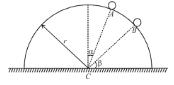Solution:

Let v be the speed of particle of B, just when it is about to loose contact.
From application of Newton's second law to the particle normal to the spherical surface.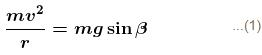Applying conservation of energy as the block moves from A to B.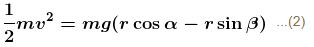Solving 1 and 2 we get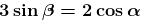The correct answer is:QUESTION: 2

A bob is attached to one end of a string other end of which is fixed at peg A. The bob is taken to a position where string makes an angle of 30° with the horizontal. On the circular path of the bob in vertical plane there is a peg ‘B’ at a symmetrical position with respect to the position of release as shown in the figure. If vc and va be the minimum speeds in clockwise and anticlockwise directions respectively, given to the bob in order to hit the peg ‘B’ then ratio vc : va is equal to :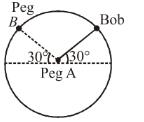Solution:

For anti-clockwise motion, speed at the highest point should be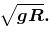Conserving energy at (1) and (2) :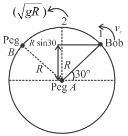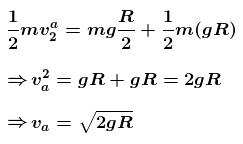For clock-wise motion, the bob must have atleast that much speed initially, so that the string must not become loose any where until it reaches the peg B.

At the initial position :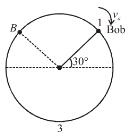vc being the initial speed in clockwise direction.
For Vc min : Put T = 0;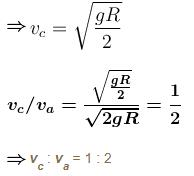The correct answer is: (1 : 2)

QUESTION: 3

The sphere at P is given a downward velocity v0 and swings in a vertical plane at the end of a rope of l = 1m  attached to a support at O. The rope breaks at angle 30° from horizontal, knowing that it can withstand a maximum tension equal to three times the weight of the sphere. Then the value of v0 will be : (g = π2 m/s)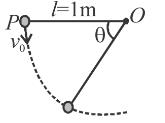Solution: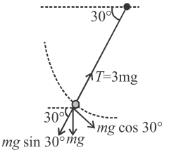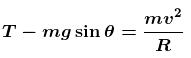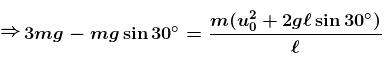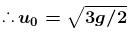The correct answer is: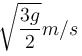QUESTION: 4

A simple pendulum is oscillating in a vertical plane. If resultant acceleration of bob of mass m at a point A is in horizontal direction, find the tangential force at this point in terms of tension T and mg.

Solution: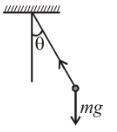When the acceleration of bob is horizontal, net vertical force on the bob will be zero.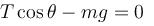The tangential force at that instant is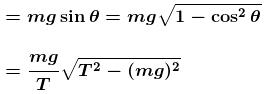The correct answer is: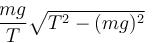QUESTION: 5

A uniform rod of mass m and length l is rotating with constant angular velocity ω about an axis which passes through its one end and perpendicular to the length of rod. The area of cross section of the rod is A and its Young’s modulus is Y. Neglect gravity. The strain at the mid point of the rod is :

Solution: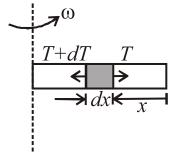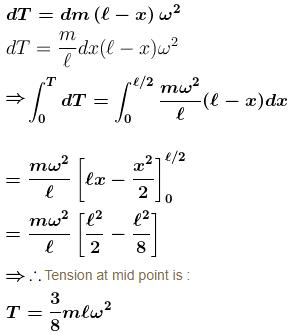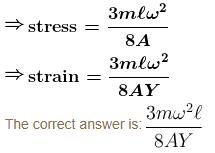QUESTION: 6

A small coin of mass 40 g is placed on the horizontal surface of a rotating disc. The disc starts from rest and is given a constant angular acceleration α = 2 rad/s2.  The coefficient of static friction between the coin and the disc is µs = 3/4 and coefficient of kinetic friction µk = 0.5. The coin is placed at a distance r = 1m from the centre of the disc. The magnitude of the resultant force on the coin exerted by the disc just before it starts slipping on the disc is :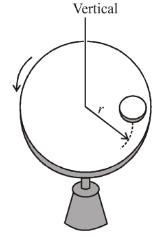Solution: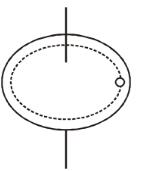The friction force on coin just before coin is to slip will be : f = µs mg
Normal reaction on the coin N = mg
The resultant reaction by disk to the coin is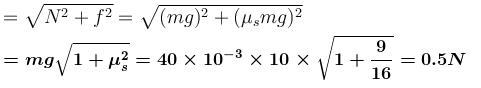The correct answer is: 0.5 N

QUESTION: 7

Objects A and B each of mass are connected by light inextensible cord. They are constrained to move on a frictionless ring in a vertical plane as shown in figure. The objects are released from rest at the positions shown. The tension in the cord just after release will be :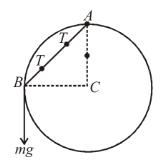Solution:

From length constraint on AB

a = b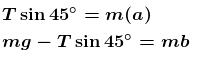mg – ma = ma
2ma = mg
a = g/2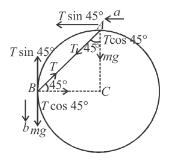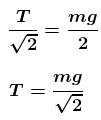The correct answer is: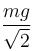QUESTION: 8

A disc of radius R has a light pole fixed perpendicular to the disc at the circumference which in turn has a pendulum of length R attached to its other end as shown in figure. The disc is rotated with a constant angular velocity ω. The string is making an angle 30° with the rod. Then the angular velocity ω of disc is :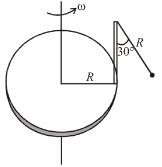Solution:

The bob of the pendulum moves in a circle of radius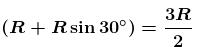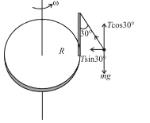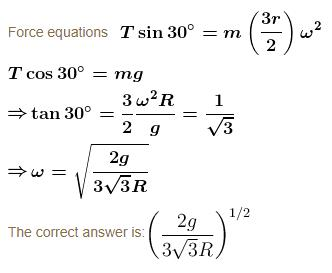QUESTION: 9

A bead of mass m is located on a parabolic wire with its axis vertical and vertex at the origin as shown in figure and whose equation is x2 = 4ay. The wire frame is fixed and the bead can slide on it without friction. The bead is released from the point y = 4a on the wire frame from rest. The tangential acceleration of the bead when it reaches the position given by y = a is :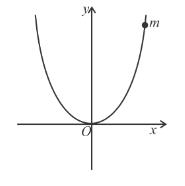Solution:

x = 4ay
Differentiating w.r.t. y, we get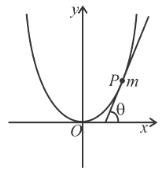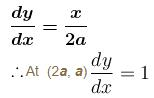the component of weight along tangential direction is mg sin θ
hence tangential acceleration is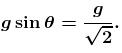The correct answer is: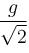QUESTION: 10

A weight W attached to the end of a flexible rope of diameter d = 0.75 cm is raised vertically by winding the rope on a reel as shown. If the reel is turned uniformly at the rate of 2 r.p.s. What is the tension in rope. The inertia of rope may be neglected.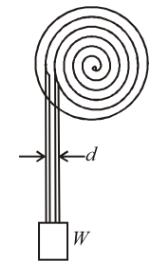Solution:

As tangential acceleration a = dv/dt = ωdr/dt
but ω = 4π and dr / dt = 1.5 (reel is turned uniformly at the rate of 2 r.p.s.)
∴ a = 6π

Now by the F.B.D. of the mass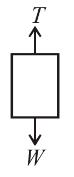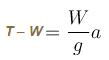∴   T = W (1 + a / g)
Put a = 6π
∴  T = 0.19W
The correct answer is: 1.019 WUse Code STAYHOME200 and get INR 200 additional OFF Use Coupon Code

Track your progress, build streaks, highlight & save important lessons and more!

Similar ContentRelated tests